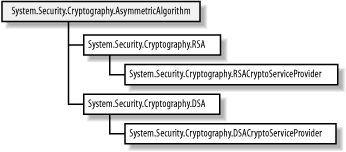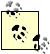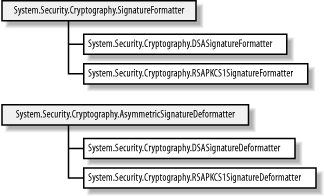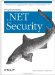# 16.2 Programming Digital Signatures

The .NET Framework groups encryption and digital signature algorithms together as subclasses of the AsymmetricAlgorithm class. Figure 16-4 depicts the .NET class hierarchy for digital signature algorithms, which differs from the encryption algorithm hierarchy only because of the addition of the signature-only DSA support.

##### Figure 16-4. The .NET Framework class hierarchy for digital signature algorithmsThe general lack of consistency between the abstract algorithm classes (RSA and DSA) and their implementation counterparts (RSACryptoServiceProvider and DSACryptoServiceProvider) means that there are several equivalent ways to accomplish signature operations, which we demonstrate in the following sections.

#### 16.2.1 Using the Abstract Class

The abstract System.Security.Cryptography.DSA class defines the CreateSignature method, which accepts a SHA-1 hash code that will be PKCS #1 formatted and signed, as the following example demonstrates (we have omitted the process of specifying the key pair to use):

`# C# // create the plaintext byte[] x_plaintext = Encoding.Default.GetBytes("Programming .NET Security"); // create the SHA-1 algorithm instance and create a hash code for the plaintext SHA1 x_sha = SHA1.Create(  ); byte[] x_hashcode = x_sha.ComputeHash(x_plaintext); // create an instance of the DSA algorithm using // the Create method in the abstract class DSA x_dsa = DSA.Create(  );          // use the CreateSignature method to sign the // SHA-1 hashcode created from the plaintext byte[] x_signature = x_dsa.CreateSignature(x_hashcode); # Visual Basic .NET ' create the plaintext Dim x_plaintext As Byte(  ) = Encoding.Default.GetBytes("Programming .NET Security") ' create the SHA-1 algorithm instance and create a hash code for the plaintext Dim x_sha As SHA1 = SHA1.Create(  ) Dim x_hashcode As Byte(  ) = x_sha.ComputeHash(x_plaintext) ' create an instance of the DSA algorithm using ' the Create method in the abstract class Dim x_dsa As DSA = DSA.Create(  ) ' use the CreateSignature method to sign the ' SHA-1 hashcode created from the plaintext Dim x_signature As Byte(  ) = x_dsa.CreateSignature(x_hashcode)`

You must create the SHA-1 hash code yourself when using the CreateSignature method. The method returns the DSA signature, expressed as an array of bytes.The DSA signature function relies on random numbers to create signatures. This means that any two signatures will be different, even when created for the same data and using the same key pair.

The VerifySignature method is the counterpart to CreateSignature, and accepts a SHA-1 hash code and the signature to verify, both expressed as an array of bytes. The following statements demonstrate how to verify a DSA signature:

`# C# // create the plaintext byte[] x_plaintext      = Encoding.Default.GetBytes("Programming .NET Security"); // define the signature to verify byte[] x_signature = new Byte[] {0x7D, 0x2B, 0xD7, 0x3D, 0x88, 0xCB, 0x1B, 0x6B,                                   0x04, 0x62, 0x95, 0xBE, 0x28, 0x59, 0x3E, 0xC5,                                   0x40, 0xDA, 0x79, 0xFE, 0x3B, 0x25, 0x08, 0x4B,                                   0x27, 0xF1, 0x31, 0x2A, 0x6F, 0x7C, 0x6E, 0x35,                                    0x45, 0x9A, 0x49, 0x4C, 0xA4, 0x5E, 0xE6, 0xA0}; // create the SHA-1 algorithm instance and // create a hash code for the plaintext SHA1 x_sha = SHA1.Create(  ); byte[] x_hashcode = x_sha.ComputeHash(x_plaintext); // create an instance of the DSA algorithm using // the Create method in the abstract class DSA x_dsa = DSA.Create(  ); // use the VerifySignature method to verify the DSA signature bool x_signature_valid = x_dsa.VerifySignature(x_hashcode, x_signature); # Visual Basic .NET ' create the plaintext Dim x_plaintext As Byte(  )= Encoding.Default.GetBytes("Programming .NET Security") ' define the signature to verify Dim x_signature As Byte(  ) = New Byte(  ) {&H7D, &H2B, &HD7, &H3D, &H88, &HCB, _                                   &H1B, &H6B, &H4, &H62, &H95, &HBE, &H28, _                                   &H59, &H3E, &HC5, &H40, &HDA, &H79, &HFE, _                                   &H3B, &H25, &H8, &H4B, &H27, &HF1, &H31, _                                   &H2A, &H6F, &H7C, &H6E, &H35, &H45, &H9A, _                                   &H49, &H4C, &HA4, &H5E, &HE6, &HA0} ' create the SHA-1 algorithm instance and ' create a hash code for the plaintext Dim x_sha As SHA1 = SHA1.Create(  ) Dim x_hashcode As Byte(  ) = x_sha.ComputeHash(x_plaintext) ' create an instance of the DSA algorithm using ' the Create method in the abstract class Dim x_dsa As DSA = DSA.Create(  ) ' use the VerifySignature method to verify the DSA signature Dim x_signature_valid As Boolean = x_dsa.VerifySignature(x_hashcode, x_signature)`

The VerifySignature method returns true if the signature can be verified and false if the signature is not valid.The abstract RSA class does not provide any methods to support digital signatures with the RSA algorithm.

#### 16.2.2 Using the Implementation Class

The RSACryptoServiceProvider and DSACryptoServiceProvider classes both define four methods related to digital signatures (DSACryptoServiceProvider implements these methods in addition to the CreateSignature and VerifySignature methods we discussed in the previous section). Table 16-2 summarizes the methods.

##### Table 16-2. Algorithm implementation signature methods

Method

Description

SignData

Creates a digital signature from the original document

SignHash

Creates a digital signature from a hash code

VerifyData

Verifies a digital signature against the original document

VerifyHash

Verifies a digital signature against a hash code

The SignData method creates a signature by generating a hash code, formatting the hash code using PKCS #1, and signing the result. The corresponding VerifyData method creates a PKCS #1-formatted hash code and uses it to verify a signature.

For the RSA algorithm, the hash codes are generated using an instance of System.Security.Cryptography.HashAlgorithm, provided as an argument to the SignData and VerifyData methods; see Chapter 13 for details of the HashAlgorithm class. For the DSA algorithm, the SHA-1 hashing algorithm is always used to generate the hash codes.

The following statements demonstrate how to use the SignData method to create a signature for a byte array, and then verify the signature using the VerifyData method:

`# C# // create the plaintext byte[] x_plaintext = Encoding.Default.GetBytes("Programming .NET Security"); // create an instance of the DSA implementation class DSACryptoServiceProvider x_dsa = new DSACryptoServiceProvider(  ); // create a signature for the plaintext byte[] x_dsa_signature = x_dsa.SignData(x_plaintext); // verify the signature, using the plaintext bool x_dsa_sig_valid = x_dsa.VerifyData(x_plaintext, x_dsa_signature); // create an instance of the RSA implementation class RSACryptoServiceProvider x_rsa = new RSACryptoServiceProvider(  ); // create an instance of the SHA-1 hashing algorithm HashAlgorithm x_sha1 = HashAlgorithm.Create("SHA1"); byte[] x_rsa_signature = x_rsa.SignData(x_plaintext, x_sha1); // verify the signature, using the plaintext bool x_rsa_sig_valid = x_rsa.VerifyData(x_plaintext, x_sha1, x_rsa_signature); # Visual Basic .NET ' create the plaintext Dim x_plaintext As Byte(  ) = Encoding.Default.GetBytes("Programming .NET Security") ' create an instance of the DSA implementation class Dim x_dsa As DSACryptoServiceProvider = New DSACryptoServiceProvider(  ) ' create a signature for the plaintext Dim x_dsa_signature As Byte(  ) = x_dsa.SignData(x_plaintext) ' verify the signature, using the plaintext Dim x_dsa_sig_valid As Boolean = x_dsa.VerifyData(x_plaintext, x_dsa_signature) ' create an instance of the RSA implementation class Dim x_rsa As RSACryptoServiceProvider = New RSACryptoServiceProvider(  ) ' create an instance of the SHA-1 hashing algorithm Dim x_sha1 As HashAlgorithm = HashAlgorithm.Create("SHA1") Dim x_rsa_signature As Byte(  ) = x_rsa.SignData(x_plaintext, x_sha1) ' verify the signature, using the plaintext Dim x_rsa_sig_valid As Boolean = x_rsa.VerifyData(x_plaintext, x_sha1, _ x_rsa_signature)`

The principal advantage of the SignData method is that the data to be signed can be read from a stream, which is useful for signing documents (which would otherwise be read into system memory). The following statements demonstrate how to use the SignData method to create a DSA signature by reading data from a stream. For this example, assume that we wish to sign a disk file called mydocument.txt:

`# C# // open the file as a stream System.IO.FileStream x_stream      = new System.IO.FileStream("mydocument.txt", System.IO.FileMode.Open); // create an instance of the DSA implementation class DSACryptoServiceProvider x_dsa = new DSACryptoServiceProvider(  ); // create a signature using the stream byte[] x_dsa_signature = x_dsa.SignData(x_stream); // close the stream x_stream.Close(  ); # Visual Basic .NET ' open the file as a stream Dim x_stream As System.IO.FileStream _ = New System.IO.FileStream("mydocument.txt", System.IO.FileMode.Open) ' create an instance of the DSA implementation class Dim x_dsa As DSACryptoServiceProvider = New DSACryptoServiceProvider(  ) ' create a signature using the stream Dim x_dsa_signature As Byte(  ) = x_dsa.SignData(x_stream) ' close the stream x_stream.Close(  )`

The SignHash and VerifyHash methods format and sign a pre-existing hash code, which is passed into the methods as an argument; the methods also require a string argument representing the identifier of the hashing algorithm that has been used to create the hash code (this is so the correct PKCS #1 algorithm ID is included in the signature). The static MapNameToOID method of the CryptoConfig class returns the ID of a hashing algorithm, so that the following statements obtain the ID for the SHA-1 algorithm:

`# C# string x_id = CryptoConfig.MapNameToOID("SHA1"); Console.WriteLine(x_id); # Visual Basic .NET Dim x_id As String = CryptoConfig.MapNameToOID("SHA1") Console.WriteLine(x_id)`

These statements produce the following output, which is a decimal representation of part of the ID that we listed in Table 16-1:

`1.3.14.3.2.26`

The following statements demonstrate how to create and verify a signature using the SignHash and VerifyHash methods and the DSA algorithm. Notice that the DSA implementation requires the ID of the hashing algorithm, even though the DSA specification demands that SHA-1 is always used:

`# C# // create the plaintext byte[] x_plaintext = Encoding.Default.GetBytes("Programming .NET Security"); // create a hash code for the plaintext, using the SHA-1 algorithm byte[] x_hashcode = HashAlgorithm.Create("SHA1").ComputeHash(x_plaintext); // create an instance of the DSA implementation class DSACryptoServiceProvider x_dsa = new DSACryptoServiceProvider(  ); // create a DSA signature using the hash code byte[] x_signature = x_dsa.SignHash(x_hashcode, CryptoConfig.MapNameToOID("SHA1")); // verify the signature bool x_sig_valid = x_dsa.VerifyHash(x_hashcode, CryptoConfig.MapNameToOID("SHA1"),     x_signature); # Visual Basic .NET ' create the plaintext Dim x_plaintext As Byte(  ) = Encoding.Default.GetBytes("Programming .NET Security") ' create a hash code for the plaintext, using the SHA-1 algorithm Dim x_hashcode As Byte(  ) = HashAlgorithm.Create("SHA1").ComputeHash(x_plaintext) ' create an instance of the DSA implementation class Dim x_dsa As DSACryptoServiceProvider = New DSACryptoServiceProvider(  ) ' create a DSA signature using the hash code Dim x_signature As Byte(  ) = x_dsa.SignHash(x_hashcode, _ CryptoConfig.MapNameToOID("SHA1")) ' verify the signature Dim x_sig_valid As Boolean = x_dsa.VerifyHash(x_hashcode, _ CryptoConfig.MapNameToOID("SHA1"), x_signature)`

#### 16.2.3 Using the Signature Formatter Classes

The .NET Framework specifies separate formatter and deformatter classes, as shown by the hierarchy in Figure 16-5. These classes format a hash code using PKCS #1, and sign the result in conjunction with the abstract and implementation classes. The functionality that these classes provide is equivalent to using the methods that we have described in the preceding sections; there is no specific advantage to using the formatter and deformatter classes.

##### Figure 16-5. The .NET Framework class hierarchy for signature formattersThe abstract AsymmetricSignatureFormatter class defines the methods described in Table 16-3. The abstract class does not specify how to format a hash code, and so implementation classes can define any formatting that is appropriate to an algorithm; both of the default .NET implementation classes use PKCS #1 formatting.

##### Table 16-3. AsymmetricSignatureFormatter members

Method

Description

SetHashAlgorithm

Specifies the name of the hashing algorithm that was used to create the hash code that will be signed; in the case of PKCS #1 formatting, the ID of the algorithm will be included in the signed data

SetKey

Specifies an instance of the asymmetric signing algorithm that will be used to create the digital signature

CreateSignature

Creates a digital signature from a hash code

The following statements demonstrate how to use the RSAPKCS1SignatureFormatter class to create a digital signature:

`# C# // create the plaintext byte[] x_plaintext = Encoding.Default.GetBytes("Programming .NET Security"); // create a hash code for the plaintext, using the SHA-1 algorithm byte[] x_hashcode = HashAlgorithm.Create("SHA1").ComputeHash(x_plaintext); // create an instance of the DSA implementation class DSACryptoServiceProvider x_dsa = new DSACryptoServiceProvider(  ); // create the signature formatter DSASignatureFormatter x_formatter = new DSASignatureFormatter(  ); // set the instance of the DSA algorithm that will sign the data x_formatter.SetKey(x_dsa); // set the name of the hashing algorithm we used to create the hash code x_formatter.SetHashAlgorithm("SHA1"); // create the formatted DSA signature byte[] x_signature = x_formatter.CreateSignature(x_hashcode); # Visual Basic .NET ' create the plaintext Dim x_plaintext As Byte(  ) = Encoding.Default.GetBytes("Programming .NET Security") ' create a hash code for the plaintext, using the SHA-1 algorithm Dim x_hashcode As Byte(  ) = HashAlgorithm.Create("SHA1").ComputeHash(x_plaintext) ' create an instance of the DSA implementation class Dim x_dsa As DSACryptoServiceProvider = New DSACryptoServiceProvider(  ) ' create the signature formatter Dim x_formatter As DSASignatureFormatter = New DSASignatureFormatter(  ) ' set the instance of the DSA algorithm that will sign the data x_formatter.SetKey(x_dsa) ' set the name of the hashing algorithm we used to create the hash code x_formatter.SetHashAlgorithm("SHA1") ' create the formatted DSA signature Dim x_signature As Byte(  ) = x_formatter.CreateSignature(x_hashcode)`

The abstract AsymmetricSignatureDeformatter is the basis for classes that verify digital signatures created by subclasses of the AsymmetricSignatureFormatter class. Table 16-4 summarizes the methods of the AsymmetricSignatureDeformatter class.

##### Table 16-4. AsymmetricSignatureDeformatter members

Method

Description

SetHashAlgorithm

Specifies the name of the hashing algorithm that will be used to verify the signature

SetKey

Specifies an instance of the asymmetric signing algorithm that will be used to verify the digital signature

VerifySignature

Verifies a digital signature

The following statements demonstrate how to verify a digital signature using the DSASignatureDeformatter class:

`# C# // create the plaintext byte[] x_plaintext = Encoding.Default.GetBytes("Programming .NET Security"); // create a hash code for the plaintext, using the SHA-1 algorithm byte[] x_hashcode = HashAlgorithm.Create("SHA1").ComputeHash(x_plaintext); // define the signature to verify byte[] x_signature = new Byte[] {0x7D, 0x2B, 0xD7, 0x3D, 0x88, 0xCB, 0x1B, 0x6B,                                  0x04, 0x62, 0x95, 0xBE, 0x28, 0x59, 0x3E, 0xC5,                                  0x40, 0xDA, 0x79, 0xFE, 0x3B, 0x25, 0x08, 0x4B,                                  0x27, 0xF1, 0x31, 0x2A, 0x6F, 0x7C, 0x6E, 0x35,                                   0x45, 0x9A, 0x49, 0x4C, 0xA4, 0x5E, 0xE6, 0xA0}; // create an instance of the DSA implementation class DSACryptoServiceProvider x_dsa = new DSACryptoServiceProvider(  ); // create the signature deformatter DSASignatureDeformatter x_deformatter = new DSASignatureDeformatter(  ); // set the instance of the DSA algorithm that will verify the signature x_deformatter.SetKey(x_dsa); // set the name of the hashing algorithm we used to create the hash code x_deformatter.SetHashAlgorithm("SHA1"); // verify the DSA signature bool x_signature_valid = x_deformatter.VerifySignature(x_hashcode, x_signature); # Visual Basic .NET ' create the plaintext Dim x_plaintext As Byte(  ) = Encoding.Default.GetBytes("Programming .NET Security") ' create a hash code for the plaintext, using the SHA-1 algorithm Dim x_hashcode As Byte(  ) = HashAlgorithm.Create("SHA1").ComputeHash(x_plaintext) ' define the signature to verify Dim x_signature As Byte(  ) = New Byte(  ) {&H7D, &H2B, &HD7, &H3D, &H88, &HCB, _                                   &H1B, &H6B, &H4, &H62, &H95, &HBE, &H28, _                                   &H59, &H3E, &HC5, &H40, &HDA, &H79, &HFE, _                                   &H3B, &H25, &H8, &H4B, &H27, &HF1, &H31, _                                   &H2A, &H6F, &H7C, &H6E, &H35, &H45, &H9A, _                                   &H49, &H4C, &HA4, &H5E, &HE6, &HA0} ' create an instance of the DSA implementation class Dim x_dsa As DSACryptoServiceProvider = New DSACryptoServiceProvider(  ) ' create the signature deformatter Dim x_deformatter As DSASignatureDeformatter = New DSASignatureDeformatter(  ) ' set the instance of the DSA algorithm that will verify the signature x_deformatter.SetKey(x_dsa) ' set the name of the hashing algorithm we used to create the hash code x_deformatter.SetHashAlgorithm("SHA1") ' verify the DSA signature Dim x_signature_valid As Boolean = _ x_deformatter.VerifySignature(x_hashcode, x_signature)`Programming .Net Security
ISBN: 0596004427
EAN: 2147483647
Year: 2005
Pages: 346

Similar book on Amazon